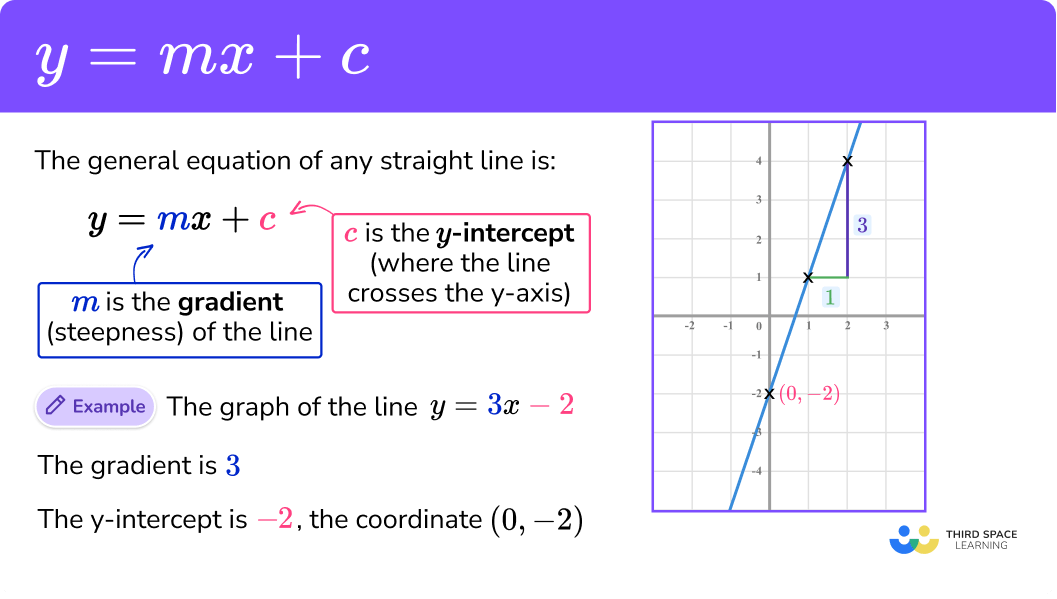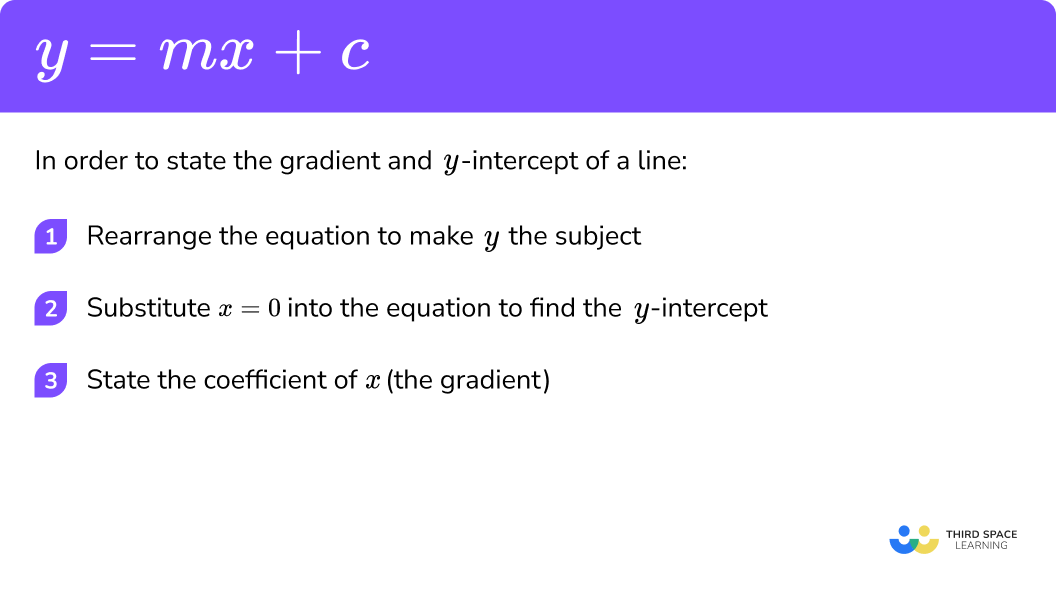# y=mx+c

Here we will learn about y = mx + c including how to recognise the gradient and y-intercept from the equation y = mx + c and rearrange an equation into the standard form of y = mx + c

There are also y = mx + c worksheets based on Edexcel, AQA and OCR exam questions, along with further guidance on where to go next if you’re still stuck.

## What is y=mx+c?

The equation y = mx + c is the general equation of any straight line where m is the gradient of the line (how steep the line is) and c is the y-intercept (the point in which the line crosses the y-axis).

y = mx + c is a linear equation and the variables x and y relate to coordinates on the line.
When we input a value for x into the equation y = mx + c, we get a result for y.
This means that x is an independent variable, and y is a dependent variable as it is determined by the value of x

E.g.

Let’s look at the line y = 2x + 1

This graph has a gradient of 2 and a y-intercept of 1, the coordinate (0,1).

• The term linear is used to describe a straight line where the variables are raised to a power no higher than 1
• If the variables are raised to a power no higher than 2 we refer to it as a quadratic
• If the variables are raised to a power no higher than 3 we refer to it as a cubic, and so on.

### What is y=mx+c?The gradient of the line tells us how steep the line is

We use the letter m to denote the gradient.

Imagine climbing a ladder. If the ladder is really close to the wall, the gradient of the ladder is really steep (you would almost be climbing vertically). Taking the base of the ladder away from the wall means that the gradient of the slope decreases reaching a lower point on the wall.

The bigger the gradient the steeper the line.

E.g.
A gradient of 2 will generate a line that is much steeper than the gradient of {\frac{1}{3}} .

See the diagram below.

The gradient of a line can be many different types of number, i.e. fractions, decimals, negatives etc.

### The y-intercept ‘c’

The y-intercept is the point of intersection between the straight line y = mx + c, and the y-axis. The y-intercept is the value of y when x = 0.

E.g.
Let’s look at the equation y = 5x + 7

To find the y intercept we substitute x = 0 into the equation.

\begin{aligned} &y=(5 \times 0)+7\\\\ &y=7 \end{aligned}

So when x = 0, y = 7, giving us the coordinate (0,7)

This is the y-intercept.

Here is a quick summary of some equations in the form y = mx + c with the gradient and y intercept highlighted.

y=mx+c

y=2x+4

y=6x-3

y=-4x

y=6-x

2

6

-4

-1

y -intercept c

4

-3

0 (the origin)

6

## How to use y=mx+c

In order to state the gradient and y-intercept of a line:

1. Rearrange the equation to make y the subject
2. Substitute x = 0 into the equation to find the y-intercept
3. State the coefficient of x (the gradient)

### Explain how to use y=mx+c### Related lessons on straight line graphs

y=mx+c is part of our series of lessons to support revision on straight line graphs. You may find it helpful to start with the main straight line graphs lesson for a summary of what to expect, or use the step by step guides below for further detail on individual topics. Other lessons in this series include:

## y = mx + c examples

### Example 1: y=mx+c form

State the gradient and y-intercept of the line y = −3x + 8.

1. Rearrange the equation to make y the subject

The equation y = −3x + 8 is already in the general form of y=mx+c so we can progress to step 2 straight away.

2Substitute x = 0 into the equation to find the y-intercept

When x = 0,

\begin{aligned} &y=(-3\times{0})+8\\\\ &y=0+8\\\\ &y=8 \end{aligned}

The y-intercept of the line y = −3x + 8 is 8, or c = 8.

3State the coefficient of x (the gradient)

The coefficient of x is −3.

The gradient of the line y = −3x + 8 is −3, or m = −3

Solution: m = −3, c = 8

### Example 2: y=mx+c form

State the gradient and y-intercept of the line y = 7 − x.

Rearrange the equation to make y the subject

Substitute x = 0 into the equation to find the y-intercept

State the coefficient of x (the gradient)

### Example 3: rearrange the equation when x is the subject

State the gradient and y-intercept of the line x = y + 10.

Rearrange the equation to make y the subject

Substitute x = 0 into the equation to find the y-intercept

State the coefficient of x (the gradient)

### Example 4: changing the subject including a fraction

State the gradient and y-intercept of the line 2x = 6y − 15.

Rearrange the equation to make y the subject

Substitute x = 0 into the equation to find the y-intercept

State the coefficient of x (the gradient)

### Example 5: changing the subject including brackets

State the gradient and y-intercept of the line 3x = 4(y − 5).

Rearrange the equation to make y the subject

Substitute x = 0 into the equation to find the y-intercept

State the coefficient of x (the gradient)

### Example 6: changing the subject including decimals

State the gradient and y-intercept of the line

$x=\frac{y+0.85}{0.2}.$

Rearrange the equation to make y the subject

Substitute x = 0 into the equation to find the y-intercept

State the coefficient of x (the gradient)

### Common misconceptions

• Incorrect inverse operation

When rearranging equations, instead of applying the inverse operation to the value being moved, the value is simply moved to the other side of the equals sign.

E.g.
y + 5 = 2x is rearranged to make y = 2x + 5

• Stating the value of m and c

A common error is to incorrectly state the values of m and c as a result of not rearranging the equation so that it is in form of y=mx+c.
Take example 3 above when x = y + 10. The gradient would be stated as 1 as this is the coefficient of x however the value of the y-intercept would be incorrectly stated as 10.

The correct answer for the y intercept is −10 as we can rearrange the equation to make y the subject. See below.

• Mixing up the gradient and the y-intercept

Take, for example, the equation y = 10 + 3x. Here, the coefficient of x is 3 and the value of y when x = 0 is 10. This means that the gradient is 3 and the y-intercept is 10, not the other way around.

### Practice y=mx+c questions

1. State the gradient, m , and y -intercept, c , for the equation

y=-5x+9

m=-5, \; c=9m=9, \; c=5m=9, \; c=-5m=-5, \; c=4The coefficient of x is -5 , so m=-5

When x=0, y=9, so c=9.

2. State the gradient, m , and y -intercept, c , for the equation

y=6-x

m=6, \; c=-1m=-1, \; c=6m=5, \; c=6m=0, \; c=5y=6-x

y=-x+6

The coefficient of x is -1 , so m=-1

When x=0, y=6, so c=6.

3. State the gradient, m , and y -intercept, c , for the equation

x=2y+5

m=\frac{1}{2}, \; c=-\frac{5}{2}m=2, \; c=5m=-2, \; c=-5m=1, \; c=7y=\frac{1}{2}x-\frac{5}{2}

The coefficient of x is \frac{1}{2} , so m=\frac{1}{2}

When x=0, y=-\frac{5}{2}, so c=-\frac{5}{2}.

4. State the gradient, m , and y -intercept, c , for the equation

3x=5y-6

m=\frac{3}{5}, \; c=\frac{6}{5}m=3, \; c=-6m=2, \; c=-1m=2y, \; c=5y=\frac{3}{5}x+\frac{6}{5}

The coefficient of x is \frac{3}{5} , so m=\frac{3}{5}

When x=0, y=\frac{6}{5}, so c=\frac{6}{5}.

5. State the gradient, m , and y -intercept, c , for the straight line

2x=3(3+y)

m=\frac{2}{3}, \; c=-3m=2, \; c=0m=6, \; c=9m=\frac{2}{3}, \; c=3y=\frac{2}{3}x-3

The coefficient of x is \frac{2}{3} , so m=\frac{2}{3}

When x=0, y=-3 so c=-3.

6. State the gradient, m , and y -intercept, c , for the equation of the line

0.5x+0.75y=0.25

m=-\frac{2}{3}, \; c=\frac{1}{3}m=0.5, \; c=0.5m=0.75,\; c=0.5m=1.5, \; c=0.5y=-\frac{2}{3}x+\frac{1}{3}

The coefficient of x is -\frac{2}{3} , so m=-\frac{2}{3}

When x=0, y=\frac{1}{3}, so c=\frac{1}{3}.

### y=mx+c GCSE questions

1.  Given that the coordinate (3,4) lies on the line y=3x+c calculate the y -intercept of the straight line.

(2 Marks)

Substitute x=3   and  y=4   into  y=3x+c   to get

4=(3\times3)+c (1mark)

(1)

4=9+c   so

c=-5 (1mark)

(1)

2.  (a)  The coordinate A=(0,2) lies on a straight line. The gradient of the line is 5 . Using this information, state the equation of the straight line.

(b)  Write the equation of a line that is parallel to a) in the form y=mx+c .

(4 Marks)

(a)

A is the y -intercept so  c=2 or when x=0, y=2   so  c=2

(1)

y=5x+c (1mark)

(1)

y=5x+2 (1mark)

(1)

(b)

y=5x+c   where
c 2 (1mark)

(1)

3.  Show m=2 for the straight line 8x-4y=12.

(3 Marks)(3)

## Learning checklist

You have now learned how to:

• reduce a given linear equation in 2 variables to the standard form y=mx+c

## Still stuck?

Prepare your KS4 students for maths GCSEs success with Third Space Learning. Weekly online one to one GCSE maths revision lessons delivered by expert maths tutors.

Find out more about our GCSE maths tuition programme.